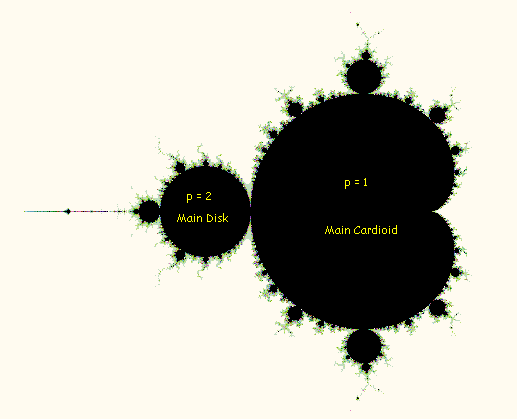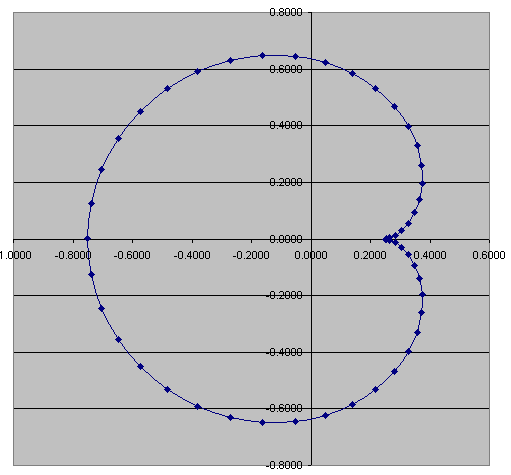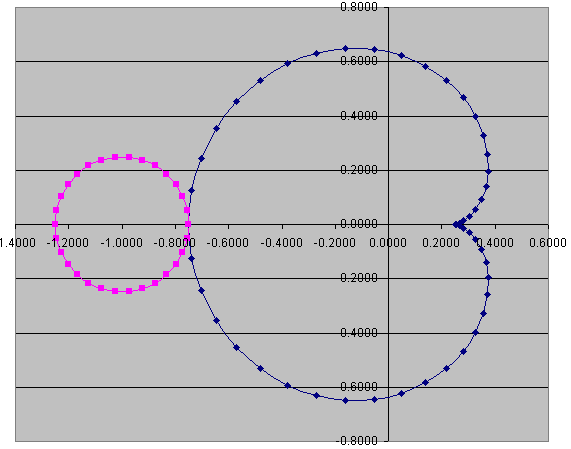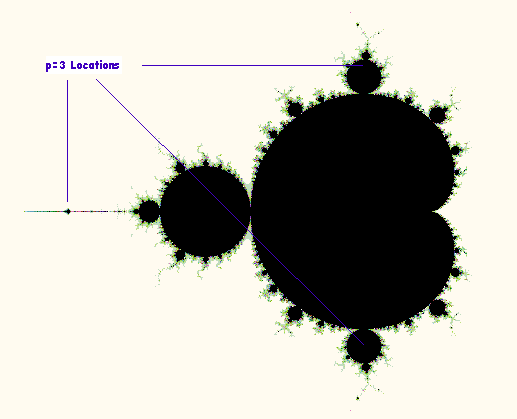[back to Cosine Kitty's math page]

## Algebraic solution of Mandelbrot orbital boundaries

Today (5 February 2005) I am working on finding exact algebraic solutions for some of the shapes of regions in the Mandelbrot Set. Specifically, I show here how to derive equations for the boundaries of the Main Cardioid and the Main Disk, as shown in Figure 1:Figure 1: The Mandelbrot Set with p=1 and p=2 regions labeled

## Mandelbrot Review

(Feel free to skip this section if you are already familiar with the mathematical definition of the Mandelbrot Set.)

We start with the value z = 0, then calculate subsequent z values by squaring z, adding c, and storing the result back as a new value of z.

To be more precise, we are forming an infinitely long list of distinct z-values. Let us refer to each value in the list using an integer subscript, like this: z[n], where n = 0, 1, 2, 3, .... We always start with

z = 0

and we calculate each subsequent value using:

z[n] = (z[n−1])2 + c
for n = 1, 2, 3, ...

We say that a complex number c is "in" the Mandelbrot Set if and only if z[n] doesn't go zooming off to infinity as n → ∞. It turns out that if z[n] goes outside a circle with a radius of 2, then future values of z will get larger and larger without bound. So another way to define the Mandelbrot Set is the set of all c such that z[n] always has a magnitude less than or equal to 2.

## The fixed-point boundary (p = 1)

It turns out that the Main Cardioid is the set of complex numbers c such that iterating z2 + c converges to a fixed point. So if you pick any complex number inside the Main Cardioid as the value of c, and iterate the formula z2 + c, you will tend toward a single complex value, which we can refer to as z[∞] = Z.

In general, if we iterate the Mandelbrot formula z2 + c a positive integer p times, and we end up back where we started, we say that we have found a periodic orbit with the value p. In mathematical notation,

z[n + p] = z[n]

So, we can restate the description of the Main Cardioid as the set of all c such that Z has a periodic orbit of p = 1. We shall call such Z a fixed point. This leads to the equation

Z2 + c = Z

Solving for c in terms of Z, we get

c = ZZ2

This tells us that for every point c that has orbital period p = 1, there must exist at least one Z such that ZZ2 = c. This is nice to know, but it doesn't help us understand what the shape of all c with period 1 looks like.

## Attractive, repelling, and indifferent points

To make further progress, we must introduce the notion of attractive, repelling, and indifferent points (see Reference ). Suppose we have any continuous and differentiable complex function f, and z = f(z). In other words, we have found a fixed point for f.

Now we ask, what happens if we feed a slightly different value through this function? Furthermore, what if we take the result and keep iterating it, by repeatedly feeding it back through the function? We don't expect the new sequence of z values to immediately repeat, but they might eventually settle down into a new fixed point. Or then again, they might not... we could end up diverging to infinity or settling into an orbital period other than 1.

In math notation, we are defining a new series of complex numbers w[n], where

w = z + ε
w[n] = f(w[n−1]); n = 1, 2, 3, ...

where we define ε (vaguely enough) as a very small but nonzero complex number.

According to the Banach Fixed Point Theorem, if |(∂/∂z) f(z)| < 1 and f(z) = z, then for sufficiently small nonzero values of ε, iterating w[n] as described above leads to convergence to a new fixed point w[∞] = W. We describe this value of z as an attractive point of the function f.

Alternatively, if the derivative of f at z has a magnitude greater than 1, the series w[n] will diverge away from z as n increases. This will happen no matter how close to zero ε gets, so long as ε ≠ 0. The series may zoom off to infinity, it may settle down into a periodic orbit away from z, or it could even be chaotic, jumping around in a seemingly random fashion, all depending on the particulars of the function f and the value z. Such values of z that push nearby values z+ε away are appropriately called repelling points.

The only possibility that remains is the marginally stable condition |(∂/∂z) f(z)| = 1. We refer to these values of z as indifferent points.

## Back to the main cardioid

Now we are sufficiently equipped to solve for the region of the Mandelbrot Set that has an orbital period of 1. We are interested in finding the boundary between attractive fixed points and repelling fixed points of f(z) = z2 + c. This boundary is simply the set of indifferent fixed points, using the equation

|(∂/∂z) (z2 + c)| = |2z| = 1

The set of complex numbers whose maginutide is 1 can be represented using Euler's Identity in terms of an angle θ as follows:

2z = e = cos(θ) + i sin(θ)
z = e / 2

Now that we have solved for the fixed-point values of z, we can plug back into the equation we derived above that relates z and c:

c = zz2
c = e/2 − (e/2)2
c = e/2 − e2iθ/4

I used a Microsoft Excel spreadsheet to plot this equation using values of θ in 50 equal steps from 0 to 2π and obtained the following graph:Figure 2: Main Cardioid plotted using c = eiθ/2 − e2iθ/4.

## The area of the Main Cardioid

The area enclosed by the Main Cardioid can be obtained by (carefully!) integrating over θ. The trick is to shift to a new origin at c = ¼ and then integrate radially. Let us define a new variable u:

u = c − ¼
u = ½ e − ¼(1 + e2iθ)

The term 1 + e2iθ causes us a problem with radial integration because we want a single integrable expression multiplied by e. It is possible to achieve this in a roundabout way using trig identities, but I found a much more direct route: Just factor out a e by brute force:

u = ½ e − ¼(e−iθ + e) e
u = ½ e − ¼(2 cos(θ)) e
u = e (1 − cos(&theta)) / 2

We can think of e as a unit vector rotating with angle θ = 0 to 2π. So the area is the integral

A = ∫0 |u|2 / 2 dθ
A = ∫0 (1 − 2cos(θ) + cos2(θ)) / 8 dθ
A = ∫0 (1 − 2cos(θ) + ½(1 + cos(2θ))) / 8 dθ
A = ∫0 (2 − 4cos(θ) + (1 + cos(2θ))) / 16 dθ
A = ∫0 (3 − 4cos(θ) + cos(2θ)) / 16 dθ
A = 3π/8 = 1.178097245096...

## The binary oscillation boundary (p = 2)

Now that we have conquered the Main Cardioid, we turn our attention to the Main Disk, which includes the set of all points c that lead to an orbital period p=2. Before we get too involved, a point of clarification is needed. We are now searching for points where p=2, which I will call binary oscillation points. We want to exclude fixed points, because we already solved for those to obtain the shape of the Main Cardioid. However, if we are not careful, we will find the fixed points again, because all fixed points are also binary oscillation points, because if f(z)=z, then trivially, f(f(z))=z also. So we need to introduce an ad hoc constraint here: we will require that the following inequality hold:

z2 + cz
or
czz2

Okay, now back to where we left off. Given

f(z) = z2 + c

we are trying to solve for

f(f(z)) = z
(z2 + c)2 + c = z

Expanding the binomial and rearranging as a quadratic in terms of c, this leads us to

c2 + (1 + 2z2)c + (z4z) = 0

Using the quadratic formula, we can find two solutions for c in terms of z:

c = ½ (−(1+2z2) ± √((1+2z2)2 − 4(z4z)))
c = ½ (−(1+2z2) ± (2z + 1))

 Solution #1 Solution #2 c = z − z2 c = −z2 − z − 1

As predicted, one of the solutions, Solution #1, corresponds to re-finding the fixed points inside the Main Cardioid; we ignore this solution, and therefore use only Solution #2.

Now we can define a new function F(z) = f(f(z)). Notice that a binary oscillation point of f is the same as a fixed point of F, so the same analytical approach we used on the Main Cardioid will work if applied to F. We use the indifference constraint as before:

(∂/∂z) F(z) = e
(∂/∂z) ((z2 + c)2 + c) = e
4z(z2 + c) = e

Substituting Solution #2 into this equation, we get:

4z(z2 + (−z2z − 1)) = e
4z(−z − 1) = e
4z(z + 1) = −e
z2 + z = −e / 4

Curiously, we can apply Solution #2 to convert z-values back into c-values, which is what we have been looking for all along:

c = z2 + z + 1
z2 + z = −c − 1

Transitively,

−c − 1 = −e / 4

And solving for c, we get the solution for the boundary of the binary oscillation region of the Mandelbrot Set:

c = ¼ e − 1

This formula describes a perfect circle, centered at −1 with a radius of ¼. The Main Disk thus has an area of π/16 = 0.196349540849.... It is interesting to note that the Main Disk's area is exactly 1/6 the area of the Main Cardioid. Adding the two regions together yields an area of 7π/16 = 1.3744467859455..., which is about 91% of the total area of the Mandelbrot Set. See also [Areas of Mandelbrot fractals].

Using Microsoft Excel again, I plotted this formula and superimposed its graph on the cardioid graph to obtain:Figure 3: Main Cardioid and Main Disk plotted together.

Compare this with Figure 1, and you see that the derivation produces a matching image with the brute force approach used to calculate the whole Mandelbrot fractal. It is remarkable that a set defined in terms of infinite iterations can have significant portions explained using exact analytic techniques.

## Grit your teeth... it's the p=3 case!

Now (8 February 2005), I'm on my way toward investigating the p=3 case. I know that this corresponds to 3 different areas on the Mandelbrot Set (after excluding the p=1 Main Cardioid solution): the large disks at the top and bottom of the cardioid, and the cardioid of the Mini-Mandelbrot whose cusp is at −1.75, as illustrated in Figure 4:Figure 4: The Mandelbrot Set with p=3 regions labeled

The problem is daunting. First, we start with the triply iterated Mandelbrot function set equal to z:

z = f(f(f(z))) = ((z2 + c)2 + c)2 + c

Expanding the binomials, we get:

z = (c + c2 + 2c3 + c4) + (4c2 + 4c3)z2 + (2c + 6c2)z4 + (4c)z6 + z8

Then, we subtract z from both sides of the equation, and rearrange as a polynomial in c:

0 = (−z + z8) + (1 + 2z4 + 4z6)c + (1 + 4z2 + 6z4)c2 + (2 + 4z2)c3 + c4

As happened in the p=2 case, the Main Cardioid is lurking here as a redundant solution. We use polynomial division by (−z + z2) + c to obtain:

0 = (1 + z + z2 + z3 + z4 + z5 + z6) + (1 + 2z + 3z2 + 2z3 + 3z4)c + (2 + z + 3z2)c2 + c3

This equation can be solved for c in terms of z, but doing so will require using the Cubic Formula, which is hard enough if the coefficients are numbers, let alone polynomial expressions! Once that is solved, we go back to the boundary constraint:

(∂/∂z) f(f(f(z))) = (8c2 + 8c3)z + (8c + 24c2)z3 + (24c)z5 + 8z7 = e
(c2 + c3)z + (c + 3c2)z3 + (3c)z5 + z7 = e / 8

Then it's a matter of plugging the solutions of c in terms of z back into this equation, expanding it all out, simplifying, and determining the shapes of the resulting curves (shudder).

That's as far as I've gotten so far. I do intend to tackle this problem, but I thought I would go ahead and publish my partially finished work in the mean time. [Stay tuned!]

## References

I am indebted to the following authors for the clues that pointed me in the right direction for solving the orbital boundaries above.
1. The Beauty of Fractals by Dr. Heinz-Otto Peitgen and Dr Peter H. Richter (ISBN 0-387-15851-0).
Chapter 2, Julia Sets and their Computergraphical Generation, gives a good explanation of superattractive, attractive, indifferent, and repelling periodic points of a general complex rational function.
2. Handbook of Mathematics and Computational Science by John W Harris and Horst Stocker (ISBN 0-387-94746-9).
Section 2.13.5, Successive Approximation, describes the Banach Fixed Point Theorem.
3. Mandelbrot Set by Eric W. Weisstein.
4. The Fractal Geometry of the Mandelbrot Set by Prof. Robert L. Devaney (Boston University).